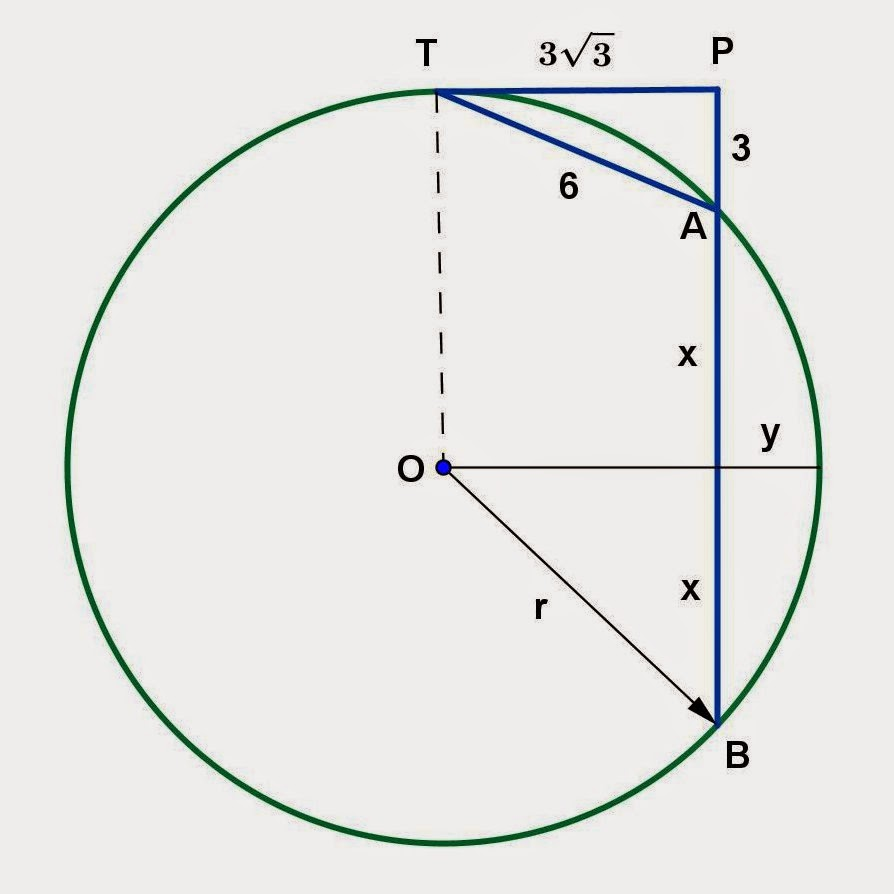Wednesday, December 17, 2014

Circle and Secant Segment Problems, 3

Category: Plane Geometry

"Published in Newark, California, USA"

PT is tangent to circle O. Secant BA is perpendicular to PT at P. If TA = 6 and PA = 3, find (a) AB, (b) the distance from O to AB, and (c) the radius of circle O.Photo by Math Principles in Everyday Life

Solution:

Consider the given figure abovePhoto by Math Principles in Everyday Life

Since PT is perpendicular to AP, then we can use Pythagorean Theorem in order to solve for the length of PT as follows

Next, let's analyze further the given figure as followsPhoto by Math Principles in Everyday Life

If you draw a line segment from point O which is perpendicular to AB, then AB will be bisected. Also, the perpendicular line is the distance of a chord or AB to the center of a circle.

If a theorem says "When two secant segments are drawn to a circle from an external point, the product of one secant segment and its external segment equals the product of the other secant segment and its external segment.", then the working equation is

Substitute the values of the line segments in order to solve for the value of x as follows

Therefore, the length of a chord is

If OT is perpendicular to PT and and PT is perpendicular to PB, then OT is parallel to PB. In this case, the distance of AB to the center of a circle is

and the radius of a circle is